Publicité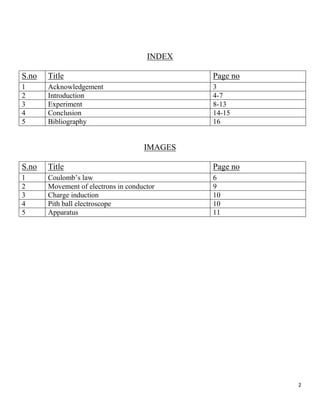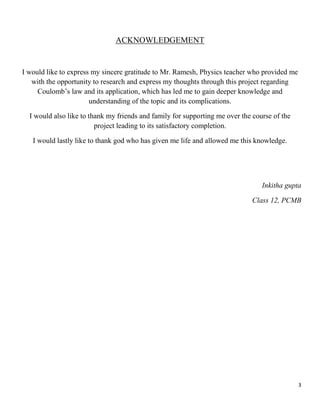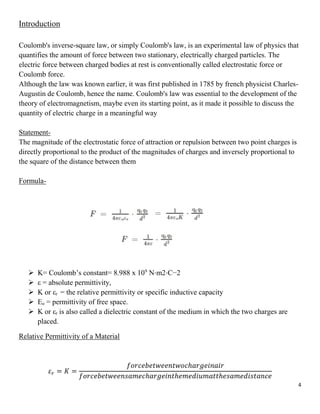Publicité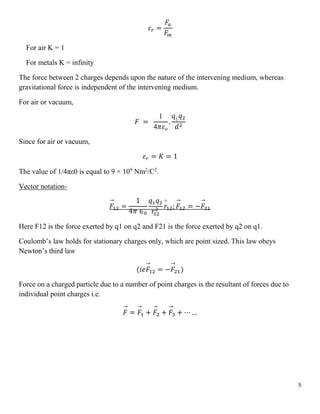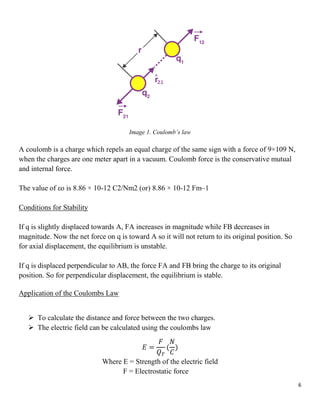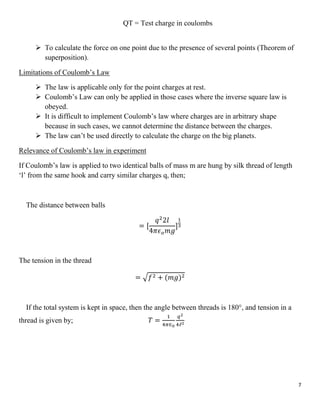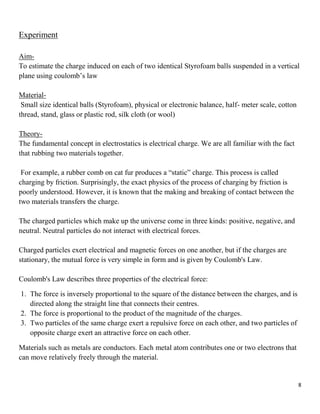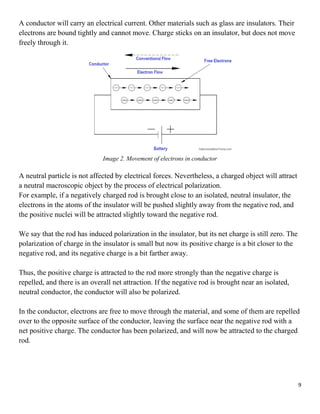Publicité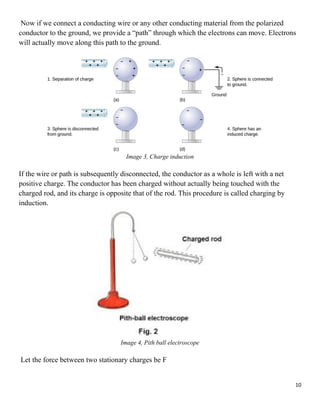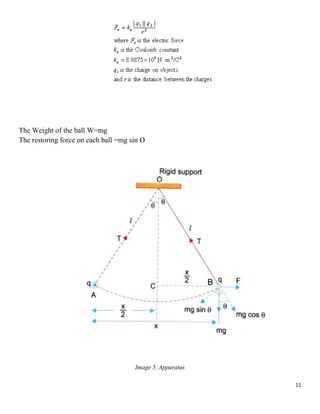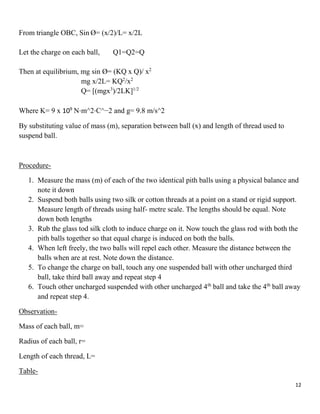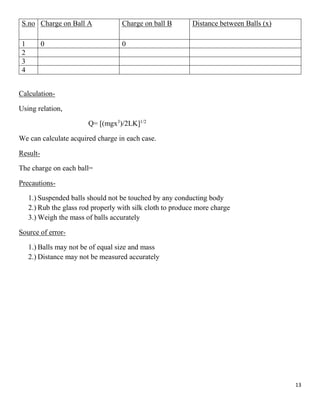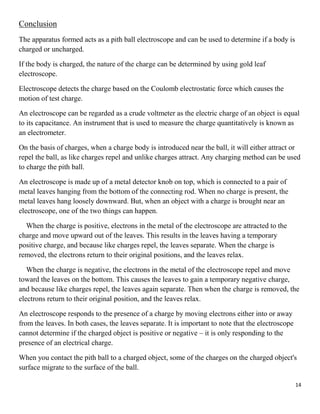Publicité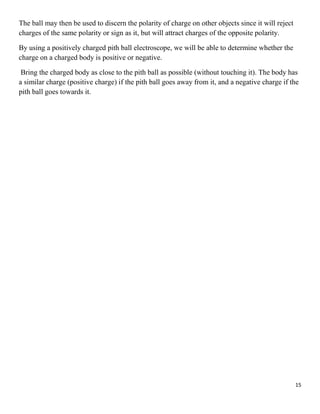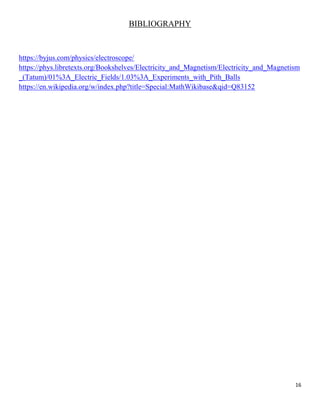Prochain SlideSharePhysics investigatory project
Chargement dans ... 3
1 sur 16
Publicité

### Physics project

1. 1 INVESTIGATORY PROJECT NAME- Inkitha gupta CLASS- XII STREAM- Science (PCMB) SUBJECT- Physics TOPIC- To estimate charge induced on two identical Styrofoam balls suspended in vertical plane by usage of Coulomb’s law.
2. 2 INDEX IMAGES S.no Title Page no 1 Acknowledgement 3 2 Introduction 4-7 3 Experiment 8-13 4 Conclusion 14-15 5 Bibliography 16 S.no Title Page no 1 Coulomb’s law 6 2 Movement of electrons in conductor 9 3 Charge induction 10 4 Pith ball electroscope 10 5 Apparatus 11
3. 3 ACKNOWLEDGEMENT I would like to express my sincere gratitude to Mr. Ramesh, Physics teacher who provided me with the opportunity to research and express my thoughts through this project regarding Coulomb’s law and its application, which has led me to gain deeper knowledge and understanding of the topic and its complications. I would also like to thank my friends and family for supporting me over the course of the project leading to its satisfactory completion. I would lastly like to thank god who has given me life and allowed me this knowledge. Inkitha gupta Class 12, PCMB
4. 4 Introduction Coulomb's inverse-square law, or simply Coulomb's law, is an experimental law of physics that quantifies the amount of force between two stationary, electrically charged particles. The electric force between charged bodies at rest is conventionally called electrostatic force or Coulomb force. Although the law was known earlier, it was first published in 1785 by french physicist Charles- Augustin de Coulomb, hence the name. Coulomb's law was essential to the development of the theory of electromagnetism, maybe even its starting point, as it made it possible to discuss the quantity of electric charge in a meaningful way Statement- The magnitude of the electrostatic force of attraction or repulsion between two point charges is directly proportional to the product of the magnitudes of charges and inversely proportional to the square of the distance between them Formula-  K= Coulomb’s constant= 8.988 x 109 N⋅m2⋅C−2  ε = absolute permittivity,  K or εr = the relative permittivity or specific inductive capacity  Εo = permittivity of free space.  K or εr is also called a dielectric constant of the medium in which the two charges are placed. Relative Permittivity of a Material 𝜀𝑟 = 𝐾 = 𝑓𝑜𝑟𝑐𝑒𝑏𝑒𝑡𝑤𝑒𝑒𝑛𝑡𝑤𝑜𝑐ℎ𝑎𝑟𝑔𝑒𝑖𝑛𝑎𝑖𝑟 𝑓𝑜𝑟𝑐𝑒𝑏𝑒𝑡𝑤𝑒𝑒𝑛𝑠𝑎𝑚𝑒𝑐ℎ𝑎𝑟𝑔𝑒𝑖𝑛𝑡ℎ𝑒𝑚𝑒𝑑𝑖𝑢𝑚𝑎𝑡𝑡ℎ𝑒𝑠𝑎𝑚𝑒𝑑𝑖𝑠𝑡𝑎𝑛𝑐𝑒
5. 5 𝜀𝑟 = 𝐹𝑎 𝐹 𝑚 For air K = 1 For metals K = infinity The force between 2 charges depends upon the nature of the intervening medium, whereas gravitational force is independent of the intervening medium. For air or vacuum, 𝐹 = 1 4𝜋𝜀o . q1 𝑞2 𝑑2 Since for air or vacuum, 𝜀𝑟 = 𝐾 = 1 The value of 1/4πε0 is equal to 9 × 109 Nm2 /C2 . Vector notation- 𝐹 → 12 = 1 4𝜋 ∈0 𝑞1𝑞2 𝑟12 2 𝑟 ^ 12; 𝐹 → 12 = −𝐹 → 21 Here F12 is the force exerted by q1 on q2 and F21 is the force exerted by q2 on q1. Coulomb’s law holds for stationary charges only, which are point sized. This law obeys Newton’s third law (𝑖𝑒𝐹 → 12 = −𝐹 → 21) Force on a charged particle due to a number of point charges is the resultant of forces due to individual point charges i.e. 𝐹 → = 𝐹 → 1 + 𝐹 → 2 + 𝐹 → 3 + ⋯ …
6. 6 Image 1. Coulomb’s law A coulomb is a charge which repels an equal charge of the same sign with a force of 9×109 N, when the charges are one meter apart in a vacuum. Coulomb force is the conservative mutual and internal force. The value of εo is 8.86 × 10-12 C2/Nm2 (or) 8.86 × 10-12 Fm–1 Conditions for Stability If q is slightly displaced towards A, FA increases in magnitude while FB decreases in magnitude. Now the net force on q is toward A so it will not return to its original position. So for axial displacement, the equilibrium is unstable. If q is displaced perpendicular to AB, the force FA and FB bring the charge to its original position. So for perpendicular displacement, the equilibrium is stable. Application of the Coulombs Law  To calculate the distance and force between the two charges.  The electric field can be calculated using the coulombs law 𝐸 = 𝐹 𝑄𝑇 ( 𝑁 𝐶 ) Where E = Strength of the electric field F = Electrostatic force
7. 7 QT = Test charge in coulombs  To calculate the force on one point due to the presence of several points (Theorem of superposition). Limitations of Coulomb’s Law  The law is applicable only for the point charges at rest.  Coulomb’s Law can only be applied in those cases where the inverse square law is obeyed.  It is difficult to implement Coulomb’s law where charges are in arbitrary shape because in such cases, we cannot determine the distance between the charges.  The law can’t be used directly to calculate the charge on the big planets. Relevance of Coulomb’s law in experiment If Coulomb’s law is applied to two identical balls of mass m are hung by silk thread of length ‘l’ from the same hook and carry similar charges q, then; The distance between balls = [ 𝑞2 2𝑙 4𝜋𝜖𝑜𝑚𝑔 ] 1 3 The tension in the thread = √𝑓2 + (𝑚𝑔)2 If the total system is kept in space, then the angle between threads is 180°, and tension in a thread is given by; 𝑇 = 1 4𝜋∈0 𝑞2 4ℓ2
8. 8 Experiment Aim- To estimate the charge induced on each of two identical Styrofoam balls suspended in a vertical plane using coulomb’s law Material- Small size identical balls (Styrofoam), physical or electronic balance, half- meter scale, cotton thread, stand, glass or plastic rod, silk cloth (or wool) Theory- The fundamental concept in electrostatics is electrical charge. We are all familiar with the fact that rubbing two materials together. For example, a rubber comb on cat fur produces a “static” charge. This process is called charging by friction. Surprisingly, the exact physics of the process of charging by friction is poorly understood. However, it is known that the making and breaking of contact between the two materials transfers the charge. The charged particles which make up the universe come in three kinds: positive, negative, and neutral. Neutral particles do not interact with electrical forces. Charged particles exert electrical and magnetic forces on one another, but if the charges are stationary, the mutual force is very simple in form and is given by Coulomb's Law. Coulomb's Law describes three properties of the electrical force: 1. The force is inversely proportional to the square of the distance between the charges, and is directed along the straight line that connects their centres. 2. The force is proportional to the product of the magnitude of the charges. 3. Two particles of the same charge exert a repulsive force on each other, and two particles of opposite charge exert an attractive force on each other. Materials such as metals are conductors. Each metal atom contributes one or two electrons that can move relatively freely through the material.
9. 9 A conductor will carry an electrical current. Other materials such as glass are insulators. Their electrons are bound tightly and cannot move. Charge sticks on an insulator, but does not move freely through it. Image 2. Movement of electrons in conductor A neutral particle is not affected by electrical forces. Nevertheless, a charged object will attract a neutral macroscopic object by the process of electrical polarization. For example, if a negatively charged rod is brought close to an isolated, neutral insulator, the electrons in the atoms of the insulator will be pushed slightly away from the negative rod, and the positive nuclei will be attracted slightly toward the negative rod. We say that the rod has induced polarization in the insulator, but its net charge is still zero. The polarization of charge in the insulator is small but now its positive charge is a bit closer to the negative rod, and its negative charge is a bit farther away. Thus, the positive charge is attracted to the rod more strongly than the negative charge is repelled, and there is an overall net attraction. If the negative rod is brought near an isolated, neutral conductor, the conductor will also be polarized. In the conductor, electrons are free to move through the material, and some of them are repelled over to the opposite surface of the conductor, leaving the surface near the negative rod with a net positive charge. The conductor has been polarized, and will now be attracted to the charged rod.
10. 10 Now if we connect a conducting wire or any other conducting material from the polarized conductor to the ground, we provide a “path” through which the electrons can move. Electrons will actually move along this path to the ground. Image 3, Charge induction If the wire or path is subsequently disconnected, the conductor as a whole is left with a net positive charge. The conductor has been charged without actually being touched with the charged rod, and its charge is opposite that of the rod. This procedure is called charging by induction. Image 4, Pith ball electroscope Let the force between two stationary charges be F
11. 11 The Weight of the ball W=mg The restoring force on each ball =mg sin Ø Image 5, Apparatus
12. 12 From triangle OBC, Sin Ø= (x/2)/L= x/2L Let the charge on each ball, Q1=Q2=Q Then at equilibrium, mg sin Ø= (KQ x Q)/ x2 mg x/2L= KQ2 /x2 Q= [(mgx3 )/2LK]1/2 Where K= 9 x 109 N⋅m^2⋅C^−2 and g= 9.8 m/s^2 By substituting value of mass (m), separation between ball (x) and length of thread used to suspend ball. Procedure- 1. Measure the mass (m) of each of the two identical pith balls using a physical balance and note it down 2. Suspend both balls using two silk or cotton threads at a point on a stand or rigid support. Measure length of threads using half- metre scale. The lengths should be equal. Note down both lengths 3. Rub the glass tod silk cloth to induce charge on it. Now touch the glass rod with both the pith balls together so that equal charge is induced on both the balls. 4. When left freely, the two balls will repel each other. Measure the distance between the balls when are at rest. Note down the distance. 5. To change the charge on ball, touch any one suspended ball with other uncharged third ball, take third ball away and repeat step 4 6. Touch other uncharged suspended with other uncharged 4th ball and take the 4th ball away and repeat step 4. Observation- Mass of each ball, m= Radius of each ball, r= Length of each thread, L= Table-
13. 13 S.no Charge on Ball A Charge on ball B Distance between Balls (x) 1 0 0 2 3 4 Calculation- Using relation, Q= [(mgx3 )/2LK]1/2 We can calculate acquired charge in each case. Result- The charge on each ball= Precautions- 1.) Suspended balls should not be touched by any conducting body 2.) Rub the glass rod properly with silk cloth to produce more charge 3.) Weigh the mass of balls accurately Source of error- 1.) Balls may not be of equal size and mass 2.) Distance may not be measured accurately
14. 14 Conclusion The apparatus formed acts as a pith ball electroscope and can be used to determine if a body is charged or uncharged. If the body is charged, the nature of the charge can be determined by using gold leaf electroscope. Electroscope detects the charge based on the Coulomb electrostatic force which causes the motion of test charge. An electroscope can be regarded as a crude voltmeter as the electric charge of an object is equal to its capacitance. An instrument that is used to measure the charge quantitatively is known as an electrometer. On the basis of charges, when a charge body is introduced near the ball, it will either attract or repel the ball, as like charges repel and unlike charges attract. Any charging method can be used to charge the pith ball. An electroscope is made up of a metal detector knob on top, which is connected to a pair of metal leaves hanging from the bottom of the connecting rod. When no charge is present, the metal leaves hang loosely downward. But, when an object with a charge is brought near an electroscope, one of the two things can happen. When the charge is positive, electrons in the metal of the electroscope are attracted to the charge and move upward out of the leaves. This results in the leaves having a temporary positive charge, and because like charges repel, the leaves separate. When the charge is removed, the electrons return to their original positions, and the leaves relax. When the charge is negative, the electrons in the metal of the electroscope repel and move toward the leaves on the bottom. This causes the leaves to gain a temporary negative charge, and because like charges repel, the leaves again separate. Then when the charge is removed, the electrons return to their original position, and the leaves relax. An electroscope responds to the presence of a charge by moving electrons either into or away from the leaves. In both cases, the leaves separate. It is important to note that the electroscope cannot determine if the charged object is positive or negative – it is only responding to the presence of an electrical charge. When you contact the pith ball to a charged object, some of the charges on the charged object's surface migrate to the surface of the ball.
15. 15 The ball may then be used to discern the polarity of charge on other objects since it will reject charges of the same polarity or sign as it, but will attract charges of the opposite polarity. By using a positively charged pith ball electroscope, we will be able to determine whether the charge on a charged body is positive or negative. Bring the charged body as close to the pith ball as possible (without touching it). The body has a similar charge (positive charge) if the pith ball goes away from it, and a negative charge if the pith ball goes towards it.
16. 16 BIBLIOGRAPHY https://byjus.com/physics/electroscope/ https://phys.libretexts.org/Bookshelves/Electricity_and_Magnetism/Electricity_and_Magnetism _(Tatum)/01%3A_Electric_Fields/1.03%3A_Experiments_with_Pith_Balls https://en.wikipedia.org/w/index.php?title=Special:MathWikibase&qid=Q83152
Publicité